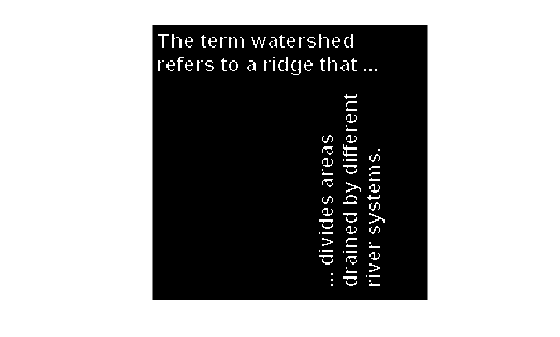Documentation

This is machine translation

Mouseover text to see original. Click the button below to return to the English version of the page.

applylut

Neighborhood operations on binary images using lookup tables

`applylut` is not recommended. Use `bwlookup` instead.

Syntax

``A = applylut(BW,lut)``

Description

example

````A = applylut(BW,lut)` performs a 2-by-2 or 3-by-3 neighborhood operation on binary image `BW` by using a lookup table, `lut`. The lookup table consists of the output values for all possible 2-by-2 or 3-by-3 neighborhoods.```

Examples

collapse all

Create the LUT.

``` lutfun = @(x)(sum(x(:))==4); lut = makelut(lutfun,2);```

Read image into the workspace and then apply the LUT to the image. An output pixel is `on` only if all four of the input pixel's neighborhood pixels are `on` .

``` BW1 = imread('text.png'); BW2 = applylut(BW1,lut);```

Show the original image and the eroded image.

` figure, imshow(BW1);`` figure, imshow(BW2);`Input Arguments

collapse all

Input image, specified as a 2-D binary image. For numeric input, any nonzero pixels are considered to be `1` (`true`).

Data Types: `single` | `double` | `int8` | `int16` | `int32` | `int64` | `uint8` | `uint16` | `uint32` | `uint64` | `logical`

Lookup table of output pixel values, specified as a 16- or 512-element vector as returned by `makelut`.

Data Types: `single` | `double` | `int8` | `int16` | `int32` | `int64` | `uint8` | `uint16` | `uint32` | `uint64` | `logical`

Output Arguments

collapse all

Output image, returned as a grayscale or binary image whose distribution of pixel values are determined by the content of the lookup table, `lut`. The output image `J` is the same size as the input image `I`.

• If all elements of `lut` are `0` or `1`, then `A` has data type `logical`.

• If all elements of `lut` are integers between 0 and 255, then `A` has data type `uint8`.

• For all other cases, `A` has data type `double`.

Data Types: `double` | `uint8` | `logical`

Algorithms

collapse all

`applylut` performs a neighborhood operation on a binary image by producing a matrix of indices into `lut`, and then replacing the indices with the actual values in `lut`. The specific algorithm used depends on whether you use 2-by-2 or 3-by-3 neighborhoods.

2-by-2 Neighborhoods

For 2-by-2 neighborhoods, `length(lut)` is 16. There are four pixels in each neighborhood, and two possible states for each pixel, so the total number of permutations is 24 = 16.

To produce the matrix of indices, `applylut` convolves the binary image `BW` with this matrix.

```8 2 4 1```

The resulting convolution contains integer values in the range [0, 15]. `applylut` uses the central part of the convolution, of the same size as `BW`, and adds 1 to each value to shift the range to [1, 16]. The function then constructs `A` by replacing the values in the cells of the index matrix with the values in `lut` that the indices point to.

3-by-3 Neighborhoods

For 3-by-3 neighborhoods, `length(lut)` is 512. There are nine pixels in each neighborhood, and two possible states for each pixel, so the total number of permutations is 29 = 512.

To produce the matrix of indices, `applylut` convolves the binary image `BW` with this matrix.

```256 32 4 128 16 2 64 8 1```

The resulting convolution contains integer values in the range [0, 511]. `applylut` uses the central part of the convolution, of the same size as `BW`, and adds 1 to each value to shift the range to [1, 512]. It then constructs `A` by replacing the values in the cells of the index matrix with the values in `lut` that the indices point to.

Compatibility Considerations

expand all

Not recommended starting in R2012b